## Valuation

A generalization of the p-adic Number first proposed by Kürschák in 1913. A valuationon a Fieldis a Function fromto the Real Numberssuch that the following properties hold for all:

1.,

2.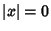Iff,

3.,

4.Implies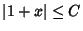for some constant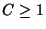(independent of).

If (4) is satisfied for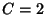, thensatisfies the Triangle Inequality,

4a.for all.

If (4) is satisfied forthensatisfies the stronger Triangle Inequality
4b..

The simplest valuation is the Absolute Value for Real Numbers. A valuation satisfying (4b) is called non-Archimedean Valuation; otherwise, it is called Archimedean.

Ifis a valuation onand, then we can define a new valuation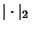by(1)

This does indeed give a valuation, but possibly with a different constant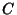in Axiom 4. If two valuations are related in this way, they are said to be equivalent, and this gives an equivalence relation on the collection of all valuations on. Any valuation is equivalent to one which satisfies the triangle inequality (4a). In view of this, we need only to study valuations satisfying (4a), and we often view axioms (4) and (4a) as interchangeable (although this is not strictly true).

If two valuations are equivalent, then they are both non-Archimedean or both Archimedean.,, and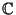with the usual Euclidean norms are Archimedean valuated fields. For any Prime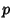, the p-adic Numberwith the-adic valuationis a non-Archimedean valuated field.

Ifis any Field, we can define the trivial valuation onby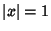for alland, which is a non-Archimedean valuation. Ifis a Finite Field, then the only possible valuation overis the trivial one. It can be shown that any valuation onis equivalent to one of the following: the trivial valuation, Euclidean absolute norm, or-adic valuation.

The equivalence of any nontrivial valuation ofto either the usual Absolute Value or to a p-adic Number absolute value was proved by Ostrowski (1935). Equivalent valuations give rise to the same topology. Conversely, if two valuations have the same topology, then they are equivalent. A stronger result is the following: Let,, ...,be valuations overwhich are pairwise inequivalent and let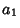,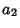, ...,be elements of. Then there exists an infinite sequence (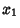,, ...) of elements ofsuch that(2)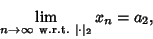(3)

etc. This says that inequivalent valuations are, in some sense, completely independent of each other. For example, consider the rationalswith the 3-adic and 5-adic valuationsand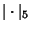, and consider the sequence of numbers given by(4)

Thenaswith respect to, butaswith respect to, illustrating that a sequence of numbers can tend to two different limits under two different valuations.

A discrete valuation is a valuation for which the Valuation Group is a discrete subset of the Real Numbers. Equivalently, a valuation (on a Field) is discrete if there exists a Real Numbersuch that(5)

The-adic valuation onis discrete, but the ordinary absolute valuation is not.

Ifis a valuation on, then it induces a metric(6)

on, which in turn induces a Topology on. Ifsatisfies (4b) then the metric is an Ultrametric. We say thatis a complete valuated field if the Metric Space is complete.

Ostrowski, A. Untersuchungen zur aritmetischen Theorie der Körper.'' Math. Zeit. 39, 269-404, 1935.